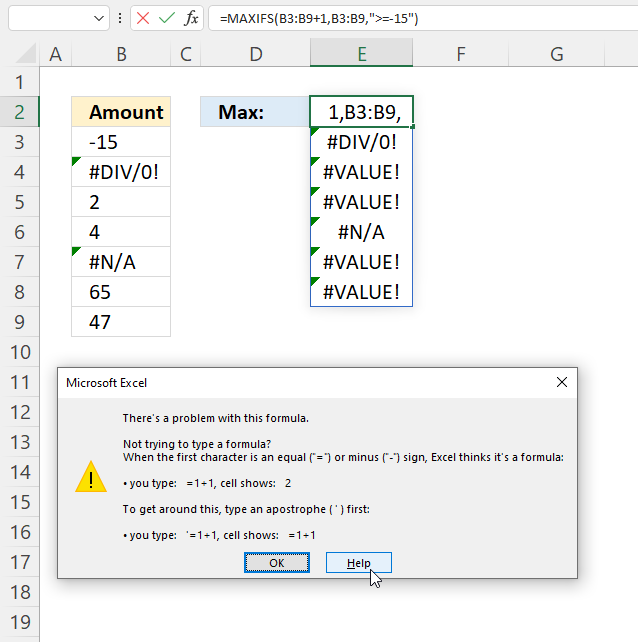Author: Oscar Cronquist Article last updated on May 05, 2022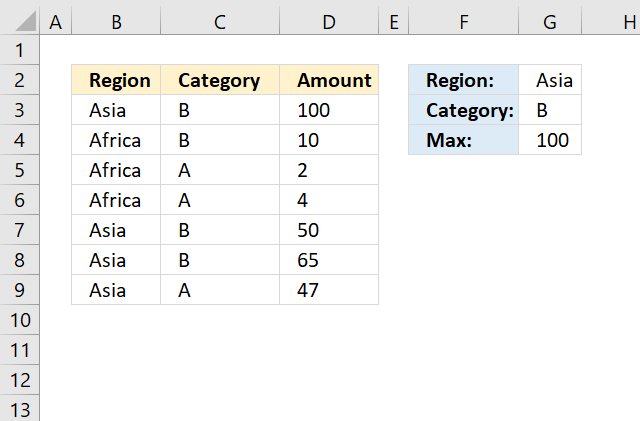The MAXIFS function returns the largest number based on a condition or criteria.

The MAXIFS function was introduced in Excel 2016. Use the MAX function and logical expressions if you have an earlier Excel version.

## 1. MAXIFS Function Syntax

MAXIFS(max_range, criteria_range1, criteria1, [criteria_range2, criteria2], ...)

You can enter up to 126 range/criteria pairs.

## 2. MAXIFS Function arguments

 max_range Required. A cell reference to numbers which the highest value will be calculated. criteria_range1 Required. The cell range you want to apply a specific condition to. criteria1 Required. The condition you want to use. [criteria_range2] Optional. Additional cell ranges. [criteria2] Optional. Additional criteria.

## 3. MAXIFS function example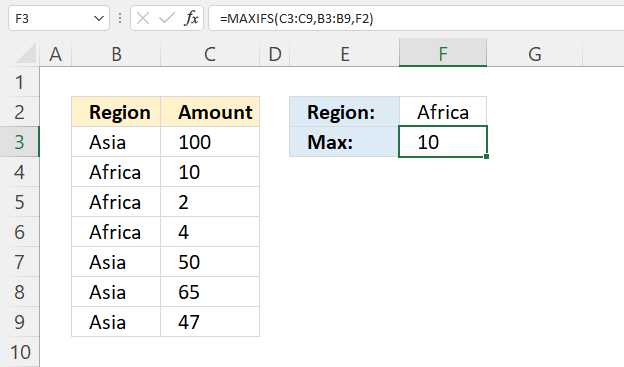The formula in cell F3 extracts the largest number in C3:C9 if the corresponding value on the same row in B3:B9 equals the condition specified in cell F2.

Formula in cell F3:

=MAXIFS(C3:C9, B3:B9, F2)

### 3.1 Explaining formula

#### Step 1 - Populate arguments

MAXIFS(max_range, criteria_range1, criteria1, [criteria_range2, criteria2], ...)

max_range - C3:C9
criteria_range1 - B3:B9
criteria1 - F2

#### Step 2 - Evaluate function

MAXIFS(B3:B9, B3:B9, F2)

becomes

MAXIFS({100; 10; 2; 4; 50; 65; 47}, {"Asia"; "Africa"; "Africa"; "Africa"; "Asia"; "Asia"; "Asia"}, "Africa")

and returns 10 in cell F3. 10 is the largest number of 10, 2, and 4.

## 4. MAXIFS function - criteriaThe formula in cell G4 in the picture above extracts the largest number (amount) that corresponds to region Asia and category B.

Formula in cell G4:

=MAXIFS(D3:D9, B3:B9, G2,C3:C9, G3)

Only three values match 100, 50, and 65. The largest of the three values is 100.

### 4.1 Explaining formula

#### Step 1 - Populate arguments

MAXIFS(max_range, criteria_range1, criteria1, [criteria_range2, criteria2], ...)

max_range - B3:B9
criteria_range1 - B3:B9
criteria1 - E2
[criteria_range2] -
[criteria2]

#### Step 2 - Evaluate function

MAXIFS(B3:B9, B3:B9, E2)

becomes

MAXIFS({100; 10; 2; 4; 50; 65; 47}, {100; 10; 2; 4; 50; 65; 47}, "<55")

and returns 50 in cell E3.

## 5. MAXIFS function - smaller than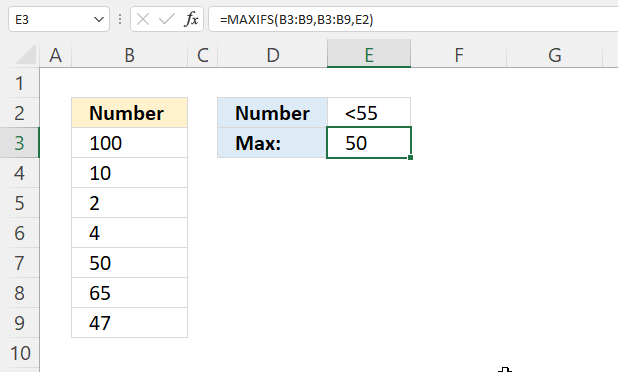The formula in cell E3 extracts the largest number in B3:B9 smaller than 55, specified in cell E2.

Formula in cell G4:

=MAXIFS(B3:B9, B3:B9, E2)

### 5.1 Explaining formula

#### Step 1 - Populate arguments

MAXIFS(max_range, criteria_range1, criteria1, [criteria_range2, criteria2], ...)

max_range - B3:B9
criteria_range1 - B3:B9
criteria1 - E2

#### Step 2 - Evaluate function

MAXIFS(B3:B9, B3:B9, E2)

becomes

MAXIFS({100; 10; 2; 4; 50; 65; 47}, {100; 10; 2; 4; 50; 65; 47}, "<55")

and returns 50 in cell E3. 50 is the largest number of 10, 2, 4, 50, and 47.

## 6. How to extract the largest number ignoring error values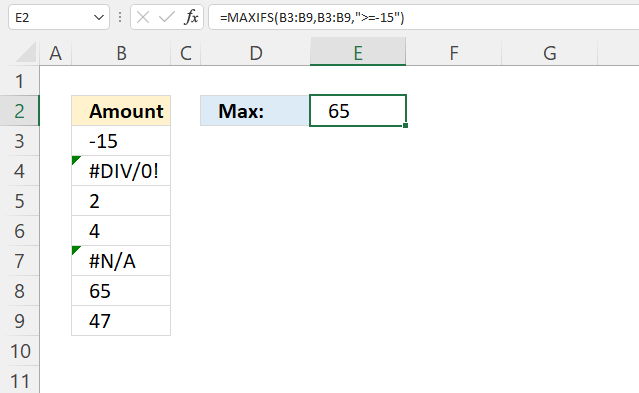The MAXIFS function ignores errors if you add a criteria pair that filters values above or equal to the smallest number in your data set.

Surprisingly, this doesn't work if you use a condition like in section 3. You need to use a smaller than or larger than sign in order to ignore error values.

Formula in cell E2:

=MAXIFS(B3:B9, B3:B9, ">=-15")

Note that this works only with cell ranges and not arrays. The image below demonstrates this problem with array values.

## 7. How to use arrays in MAXIFS function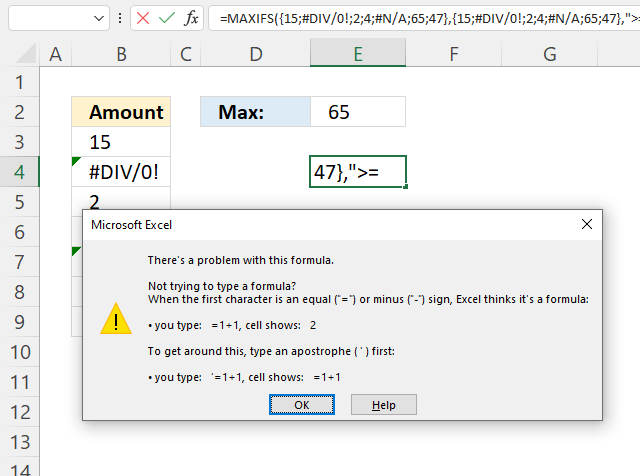In fact, the MAXIFS function doesn't accept anything else than cell references as max_range and criteria_range.

I recommend the IFERROR function and the regular MAX function to filter out error values.

Example array formula:

=MAX(IFERROR(B3:B9, ""))

### 7.1 How to enter an array formula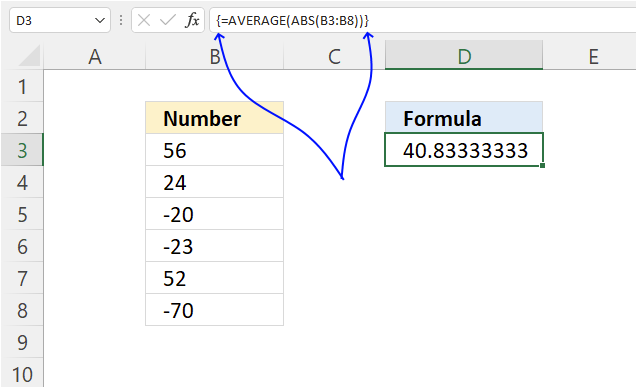The image above shows leading and trailing curly brackets. They appear automatically when you follow the steps below.

1. Copy the array formula above.
2. Double press with the left mouse button on cell D3, a prompt appears.
3. Paste it to cell C3, shortcut keys are CTRL + v.
4. Press and hold CTRL + SHIFT keys simultaneously.
5. Press Enter once.
6. Release all keys.

The formula bar shows a beginning and ending curly bracket, don't enter these characters yourself.

### 7.2 Explaining array formula

#### Step 1 - Replace error values with blanks

The IFERROR function can replace error values with a given value.

IFERROR(valuevalue_if_error)

IFERROR(B3:B9, "")

becomes

IFERROR({-15; #DIV/0!; 2; 4; #N/A; 65; 47}, "")

and returns

{-15; ""; 2; 4; ""; 65; 47}

#### Step 2 - Get the largest number

The MAX function returns the largest number ignoring text and blank values.

MAX(IFERROR(B3:B9, ""))

becomes

MAX({-15; ""; 2; 4; ""; 65; 47})

and returns 65.

## 8. Can you use other functions in the MAXIFS function?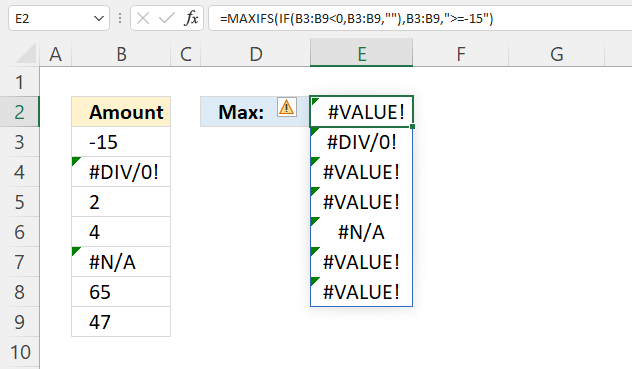No, the picture above shows a nested MAXIFS function. It contains an IF function in the first argument and it returns a bunch of error values in Excel 365.